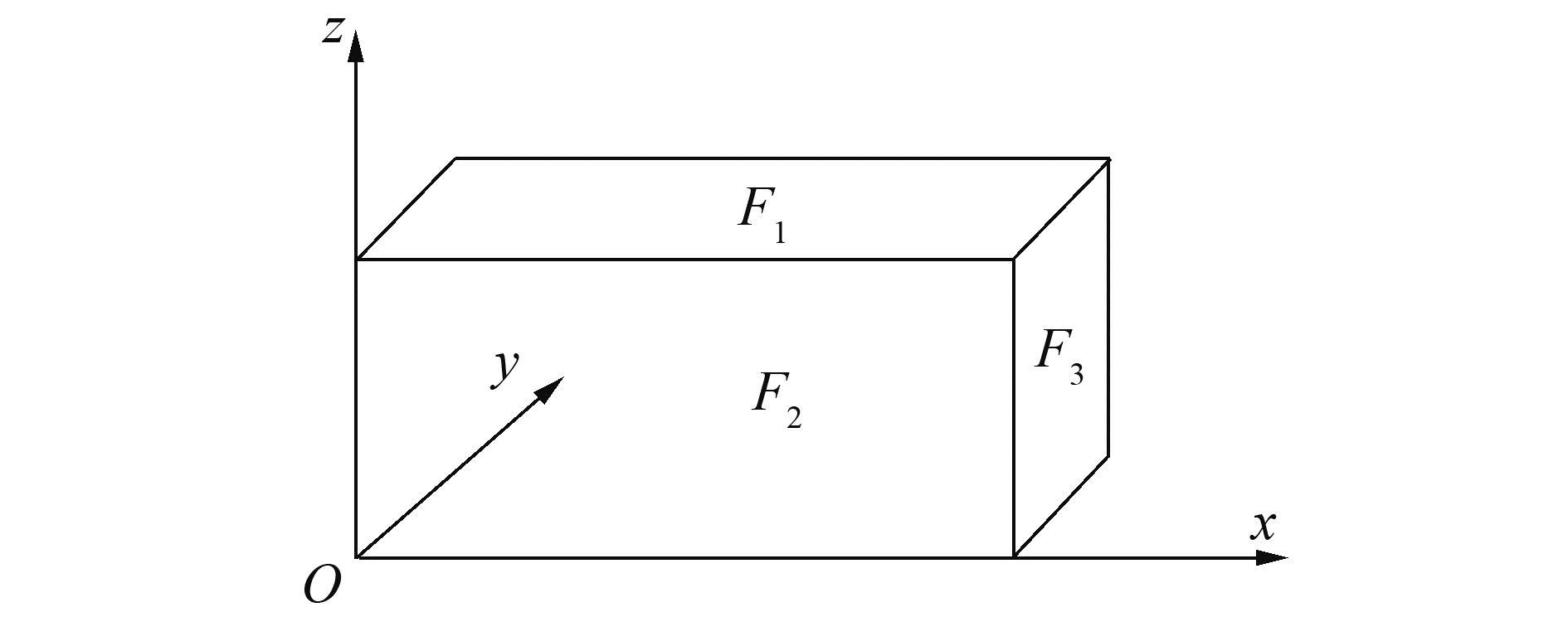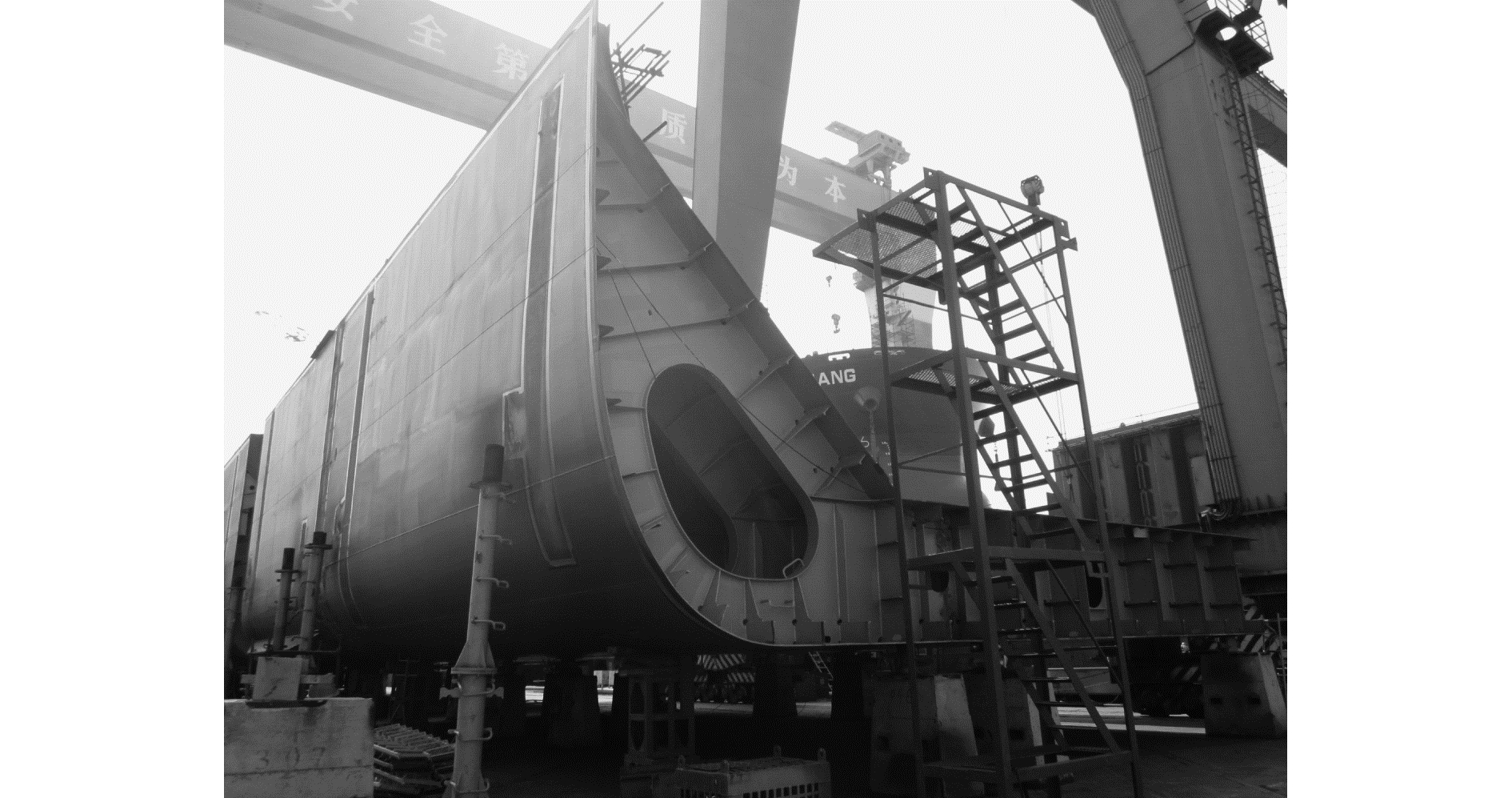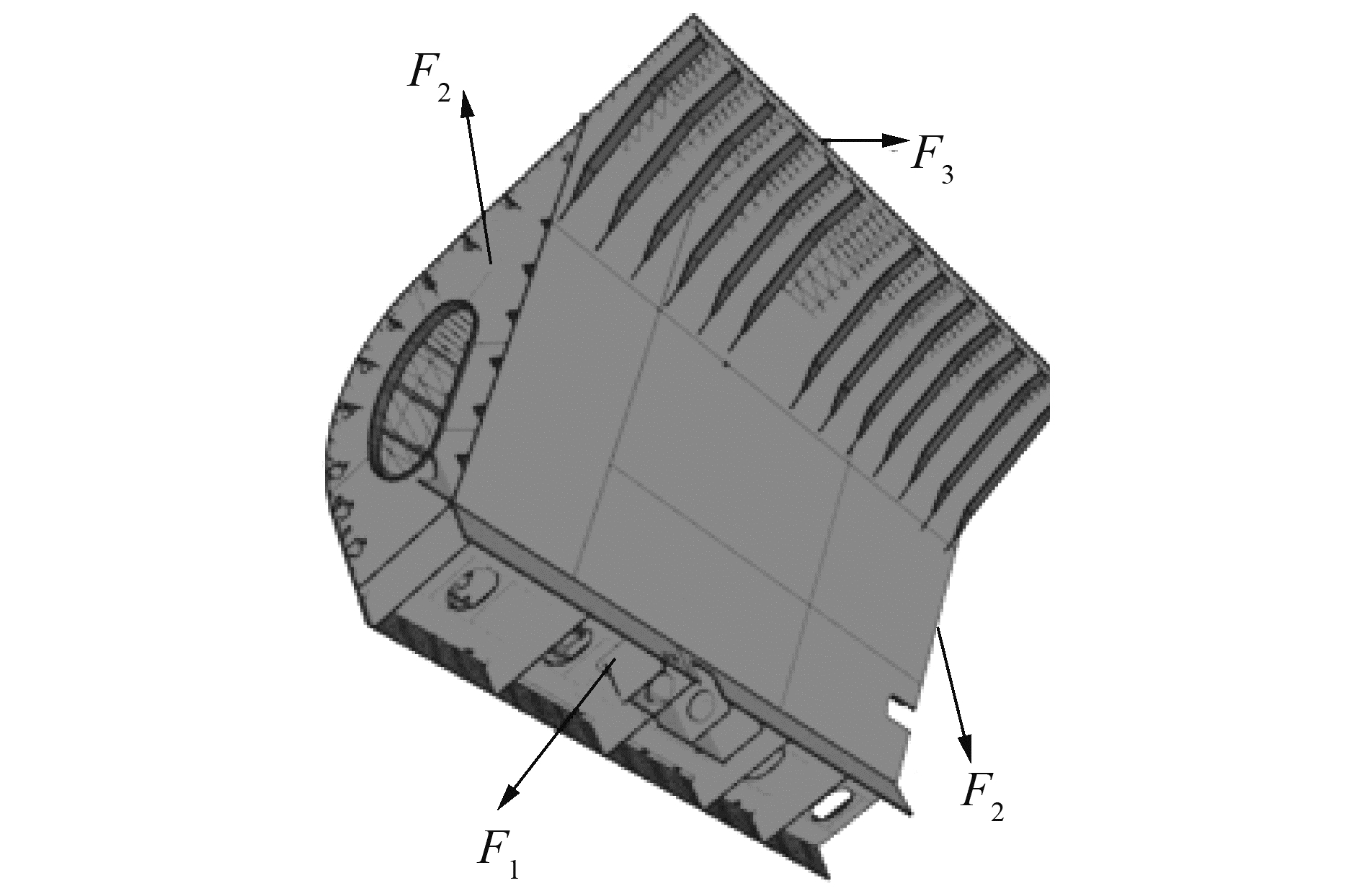﻿ 考虑安装工艺的多特征模型匹配定位
«上一篇文章快速检索 高级检索

 哈尔滨工程大学学报2019, Vol. 40Issue (8): 1393-1398  DOI: 10.11990/jheu.2018071230

引用本文YANG Guiqiang, LIU Yujun, LI Rui, et al. Matching localization of multi-feature considering the installation process[J]. Journal of Harbin Engineering University, 2019, 40(8), 1393-1398. DOI: 10.11990/jheu.201807123.文章历史

1. 大连理工大学 船舶工程学院, 辽宁 大连 116024;
2. 大连理工大学 工业装备结构分析国家重点实验室, 辽宁 大连 116024;
3. 大连理工大学 高新船舶与深海开发装备协同创新中心, 辽宁 大连 116024

Matching localization of multi-feature considering the installation process
YANG Guiqiang 1, LIU Yujun 1,2, LI Rui 1, WANG Ji 1,2,3
1. School of Naval Architecture, Dalian University of Technology, Dalian 116024, China;
2. State Key Laboratory of Structural Analysis for Industrial Equipment, Dalian University of Technology, Dalian 116024, China;
3. Collaborative Innovation Center for Advanced Ship and Deep-Sea Exploration(CISSE), Dalian University of Technology, Dalian 116024, China
Abstract: The matching and localization precision of hull blocks directly affects geometrical error evaluation in hull block construction. The evaluation parameter plays an important role in the precision management of hull blocks. Meeting the multifeature model localization requirement through the traditional pose-matching method is difficult. The sequential matching and localization of the multifeature model were studied in accordance with the error distribution optimization theory of data-matching technology to identify the best pose matching of hull blocks and to provide a reasonable method for the evaluation for construction precision. In this study, the installation process was considered. Feature sections were positioned in accordance with differences in regional precision. The iterative solution method based on model transformation theory and that integrates regional tolerance constraints was applied. It is a practical technology used for precision size monitoring in shipbuilding. Theoretical and experimental results confirmed the validity and rationality of the method related to the traditional least-squares method and showed that the proposed method can provide a quantitative data basis for the verification of models and subsequent assembly.
Keywords: hull block    accuracy control    multifeature model    region precision    matching    sequential localization    error distribution    quantitative analysis

1 多特征模型的依次定位方法 1.1 配准约束强度分析

 $f=\min \sum\limits_{i=1}^{m}\left\|\boldsymbol{s}_{i}-\left(\boldsymbol{R} p_{i}+\boldsymbol{T}\right)\right\|_{2}^{2}$ (1)

 $\mathit{\boldsymbol{R}} = \left[ {\begin{array}{*{20}{c}} {c{\theta _z}c{\theta _y}}&{c{\theta _z}s{\theta _y}s{\theta _x} - s{\theta _z}c{\theta _x}}&{c{\theta _z}s{\theta _y}c{\theta _x} + s{\theta _z}s{\theta _x}}\\ {{\rm{s}}{\theta _z}{\rm{c}}{\theta _y}}&{{\rm{s}}{\theta _z}{\rm{s}}{\theta _y}{\rm{s}}{\theta _x} + {\rm{c}}{\theta _z}{\rm{c}}{\theta _x}}&{{\rm{s}}{\theta _z}{\rm{s}}{\theta _y}{\rm{c}}{\theta _x} - {\rm{c}}{\theta _z}{\rm{s}}{\theta _x}}\\ { - {\rm{s}}{\theta _y}}&{{\rm{c}}{\theta _y}{\rm{s}}{\theta _x}}&{{\rm{c}}{\theta _y}{\rm{c}}{\theta _x}} \end{array}} \right]$ (2)
 $\mathit{\boldsymbol{t}} = {\left[ {\begin{array}{*{20}{l}} {{t_x}}&{{t_y}}&{{t_z}} \end{array}} \right]^{\rm{T}}}$ (3)

 $\mathit{\boldsymbol{z}} = {\left[ {\begin{array}{*{20}{l}} {{t_x}}&{{t_y}}&{{t_z}}&{{\theta _x}}&{{\theta _y}}&{{\theta _z}} \end{array}} \right]^{\rm{T}}}$ (4)

 $\begin{array}{l} {\varepsilon _i} = {V_i} \cdot \mathit{\boldsymbol{d}}(\mathit{\boldsymbol{z}}) = \left[ {\begin{array}{*{20}{c}} {{n_x}}&{{n_y}}&{{n_z}{{\left( {{p_i} \times {\mathit{\boldsymbol{n}}_i}} \right)}^{\rm{T}}}] \cdot \mathit{\boldsymbol{d}}(\mathit{\boldsymbol{z}})}& = \end{array}} \right.\\ \left[ {{n_x}{n_y}{n_z}{{\left( {{p_i}{\mathit{\boldsymbol{n}}_i}} \right)}^{\rm{T}}}} \right] \cdot \mathit{\boldsymbol{d}}(\mathit{\boldsymbol{z}}) \end{array}$ (5)

 $\begin{array}{l} E = \sum {\varepsilon _i^2} = {(\mathit{\boldsymbol{d}}(\mathit{\boldsymbol{z}}))^{\rm{T}}}\left( {\sum\limits_{i = 1}^m {\mathit{\boldsymbol{V}}_i^{\rm{T}}} {\mathit{\boldsymbol{V}}_i}} \right)\mathit{\boldsymbol{d}}(\mathit{\boldsymbol{z}}) = \\ {(\mathit{\boldsymbol{d}}(\mathit{\boldsymbol{z}}))^{\rm{T}}}\mathit{\boldsymbol{ \boldsymbol{\varPsi} d}}(z) \end{array}$ (6)

1.2 主元方向约束的配准变换

1) 将变换参数zj1从基本单位矢量ek(k=1, 2, …, 6)变换到按降序排列的特征值所对应的特征基$q_1^{j0}, q_2^{j0}, \cdots , q_6^{j0}$下，得到zqj1

2) 将zqj1的前r个分量置0，得到$\tilde z_q^{j1}$；即$\tilde z_q^{j1} = \mathit{\boldsymbol{ \boldsymbol{\varLambda} }} \cdot \mathit{\boldsymbol{z}}_q^{j1}$，其中Λ为主对角矩阵，前r个主对角为0，其余为1；

3) 将$\tilde z_q^{j1}$逆变换到单位基ek(k=1, 2, …, 6)下，得到约束下的配准变换${{\tilde z}^{j1}}$，即：

 ${{\tilde z}^{j1}} = {\mathit{\boldsymbol{Q}}^{ - 1}}\left( {\mathit{\boldsymbol{ \boldsymbol{\varLambda} }}\left( {\mathit{\boldsymbol{Q}}{z^{j1}}} \right)} \right)$ (7)

1.3 依次匹配定位算法

1) 当j=1时，设ΛQ均为单位矩阵；

2) 将pij－1Fj区域上数据与对应的工艺数模点应用非线性配准模型得到变换参数zj，通过主元约束配准变换公式计算${{\tilde z}^j}$，并将${{\tilde z}^j}$作用到所有待配准点得到新的测量数据pij

3) 对pij中的$F_{k(1 \leqslant k \leqslant j, k \in \bf{N})}$特征面上数据进行主元约束强度分析，获得按降序排列的特征值λ1j, λ2j, …, λ6j和对应的特征向量q1j, q2j, …, q6j。若大于0特征值的个数r < 6，将Λ的前r个主对角元素值置0，并更新矩阵Qq1j, q2j, …, q6j，转4)；若r=6，各方向均处于约束状态，程序结束；

4) 当j=j+1，且jl，转2)；当j=j+1，若j>l时，程序结束。

2 多特征模型配准试验 2.1 长方体模型配准试验Download: 图 1 多特征配准模型 Fig. 1 Registration of multi-feature model表 1 依次定位及ICP算法位姿定位结果比对 Table 1 Comparison of results of in-order localization and ICP algorithm

2.2 船体分段模型配准试验Download: 图 2 现场船体分段 Fig. 2 Hull blockDownload: 图 3 三维工艺模型 Fig. 3 3-D process model表 2 工艺模型中精度管理点的设计坐标 Table 2 Coordinates of precision management points in process model表 3 全站仪测量的管理点坐标 Table 3 Coordinates of management points measured by total station表 4 粗配准后的测量点坐标 Table 4 Coordinates of measurement points after rough registration表 5 依次配准后测量点坐标 Table 5 Coordinates of measurement points after in-order registration表 6 ICP算法配准变换后测量点坐标 Table 6 Coordinates of measurement points after registration with ICP algorithm表 7 依次配准后测量点偏差 Table 7 Errors of measurement points after in-order registration表 8 ICP算法配准后测量点偏差 Table 8 Errors of measurement points after registration with ICP algorithm表 9 船体分段测量数据配准结果比对 Table 9 Comparison of measurement data registration results for hull block
3 结论

1) 有区别对待低精度区域数据和高精度区域数据对配准位姿定位结果的影响强度。在对同一配准方向均有约束时，只保留影响强度高的配准约束。

2) 将主元约束引入到优化模型中，充分利用高精度特征数据作用，抑制低精度特征数据的不利影响，使船体分段高精度断面有高的配准准确度，低精度断面的配准准确度低，得到与实际建造过程相符合的精度评价。

  SU Fa. The research of optical 3D measuring precision influencing factor in reverse engineering[J]. Applied mechanics and materials, 2010, 33: 157-162. DOI:10.4028/www.scientific.net/AMM.33 (0)  AMEDDAH H, ASSAS M. Bio-CAD reverse engineering of free-form surfaces by planar contours[J]. Computer-aided design and applications, 2011, 8(1): 37-42. DOI:10.3722/cadaps.2011.37-42 (0)  HORN B K P. Closed-form solution of absolute orientation using unit quaternions[J]. Journal of the optical society of america A, 1987, 4(4): 629-642. DOI:10.1364/JOSAA.4.000629 (0)  刘涛, 王宗义, 金鸿章, 等. 船体分段三维数字化测量数据配准[J]. 哈尔滨工程大学学报, 2010, 31(3): 345-349. LIU Tao, WANG Zongyi, JIN Hongzhang, et al. The data registration of 3-D digital measurement for hull blocks[J]. Journal of Harbin Engineering University, 2010, 31(3): 345-349. (0)  管官, 申玫, 林焰, 等. 船体分段测量点数据与CAD模型自动匹配方法研究[J]. 哈尔滨工程大学学报, 2012, 33(5): 580-584. GUAN Guan, SHEN Mei, LIN Yan, et al. Methods of automatically matching measurment points data with CAD model for hull blocks[J]. Journal of Harbin Engineering University, 2012, 33(5): 580-584. DOI:10.3969/j.issn.1006-7043.201109020 (0)  李文亮. 四元数矩阵[M]. 长沙: 国防科技大学出版社, 2002: 10-62. (0)  UMEYAMA S. Least-squares estimation of transformation parameters between two point patterns[J]. IEEE transactions on pattern analysis and machine intelligence, 1991, 13(4): 376-380. DOI:10.1109/34.88573 (0)  BESL P J, MCKAY N D. A method for registration of 3-D shapes[J]. IEEE Transactions on Pattern Analysis and Machine Intelligence, 1992, 14(2): 239-256. DOI:10.1109/34.121791 (0)  王欣, 张明明, 于晓, 等. 应用改进迭代最近点方法的点云数据配准[J]. 光学精密工程, 2012, 20(9): 2068-2077. WANG Xin, ZHANG Mingming, YU Xiao, et al. Point cloud registration based on improved iterative closest point method[J]. Optics and precision engineering, 2012, 20(9): 2068-2077. (0)  谭高山, 张丽艳, 刘胜兰, 等. 基于M-估计的线性化稳健配准算法研究[J]. 农业机械学报, 2015, 46(4): 360-364, 343. TAN Gaoshan, ZHANG Liyan, LIU Shenglan, et al. Linearized robust registration algorithm based on M-estimation[J]. Transactions of the Chinese society for agricultural machinery, 2015, 46(4): 360-364, 343. (0)  陈善勇, 李圣怡, 戴一帆. 考虑特征差异的多特征工件依次定位[J]. 机械工程学报, 2003, 39(7): 13-17. CHEN Shanyong, LI Shengyi, DAI Yifan. Multi-feature workpiece inorder localization considering the differences between features[J]. Chinese journal of mechanical engineering, 2003, 39(7): 13-17. DOI:10.3321/j.issn:0577-6686.2003.07.003 (0)  SIMON D A. Fast and accurate shape-based registration[D]. Pittsburgh, Pennsylvania: Carnegie Mellon University, 1996. (0)  刘胜兰, 张丽艳, 王晓飞. 一种考虑区域精度差异的模型配准方法[J]. 机械工程学报, 2013, 49(13): 139-144. LIU Shenglan, ZHANG Liyan, WANG Xiaofei. A shape registration method considering the regional difference in precision[J]. Journal of mechanical engineering, 2013, 49(13): 139-144. (0)  段黎明, 王侃, 陈中. 工业CT重建模型与原始CAD模型粗配准的改进[J]. 计算机集成制造系统, 2013, 19(4): 673-679. DUAN Liming, WANG Kan, CHEN Zhong. Improved rough registration algorithm between industrial CT reconstruction model and original CAD model[J]. Computer integrated manufacturing systems, 2013, 19(4): 673-679. (0)  LI Zexiang, GOU Jianbo, CHU Yunxian. Geometric algorithms for workpiece localization[J]. IEEE transactions on robotics and automation, 1998, 14(6): 864-878. DOI:10.1109/70.736771 (0)  GOU Jianbo, CHU Yunxian, XIONG Zhenhua, et al. A geometric method for computation of datum reference frames[J]. IEEE transactions on robotics and automation, 2000, 16(6): 797-806. DOI:10.1109/70.897790 (0)